# Introduction to Loops in Matlab

The purpose of this article is to introduce Loops in Matlab. The original purpose of MATLAB was to develop mathematical simulations, and it has been very useful for manipulating static numerical data in vectors and matrices ever since. However, it is now capable of reading data from many sources, including flat files, databases, cloud storage, and live financial data feeds. Data science is one of the most popular areas where MATLAB is being applied, and it is used in many different industries and can also get matlab assignment help.

A ‘loop’ statement is one that repeatedly executes an instruction in all computer languages. LOOPS, in particular, allows coders to write shorter codes by bypassing repetitions of repetitive codes into conditional loops. As a general principle, a loop is a series of instructions that are repeated every time a certain condition is met or until the condition is reached.

## Programming loops in MATLAB

While and for loops are used in MATLAB. Additionally, you can nest loops within loops, allowing you to use either for or while loops.

### FOR Loop

If a set of instructions is to be executed a certain number of times, the FOR loop is used. Accordingly, it is used to run code repeatedly depending on whether a condition is met. In the FOR loop, the initialization of the loop is the condition that is defined at the beginning. In MATLAB, the FOR loop syntax is as follows:

Syntax:

When index = value

program statements

… ;

end

There are several ways in which values can be expressed:

#### 1. Initialvalue:endvalue

for a = 10:20

fprintfl (‘value of a: %d\n’, a);

end

Output: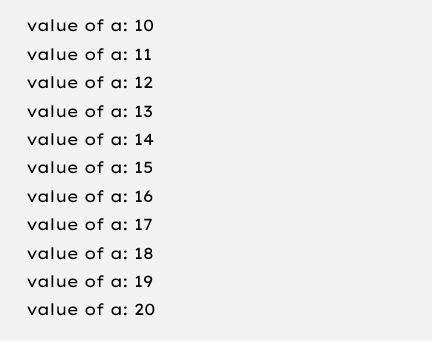#### 2. Initialvalue:step:endvalue:

A positive value step will increase the index with each iteration, while a negative value step will decrease the index.

Code:

for a = 1.0: -0.1: 0.0

disp(a);

end

Output: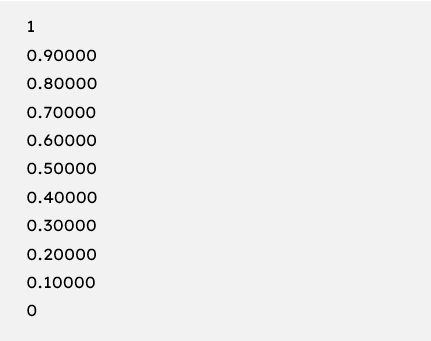#### 3. valArray

A column vector index is generated by the loop n times for each iteration of the array valArray. Allows you can input any MATLAB data type into the valArray, including cell arrays, strings, and structures.

Output: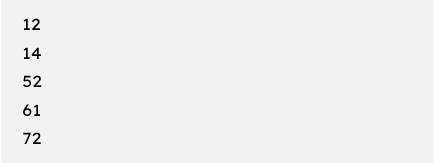### WHILE Loop

As long as the specified condition is true, the statements in the while loop will be repeatedly executed. The syntax for the while loops in MATLAB can be found below.

Syntax:

while <condition>

…allows

program statement

… ;

end

When the result does not contain nulls (either logical or numerical), it is true. Otherwise, it is false. In the while loop initialization, the comparative value for the condition is set before beginning the while loop.

The structure of the while loop changes considerably when compared to the first for loop example. Let us consider the same condition we used in the first, loop example.

Code:

a = 10;

while (a < 20)

fprintf(‘value of a: %d\n’, a);

a = a+1;

end

‘A’ is set to its initial value before starting a loop, and the loop repeats as long as the value of ‘a’ is less than 20. ‘A’ is then incrementally increased by 1, and the loop is repeated until a < 20. As opposed to the for-loop result, this is how the result looks.

Output: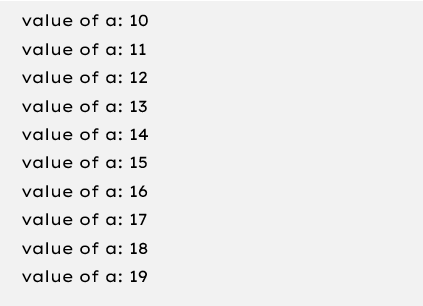Hence, the output of the loop shows only the values of a between 10 and 19. a = 20 is not considered True, and thus the code inside the loop is not executed.

### NESTED Loops

A compound statement is by using two loops inside each other. For example, a while loop can be nested within a for loop, allows or a while loop can be nested within a while loop. The resulting loop must satisfy two conditions.  Allows is means that the outer loop condition is checked first, and if True, the condition in the second nested loop is also tested.

To illustrate this, let’s look at a program that shows all prime numbers between 1 and 100.

Code:

for i = 2:100

for j = 2:100

if (~mod(i,j))

break;

end

end

if (j > (i/j))

fprintf(‘%d is prime\n’, i);

end

end

Output: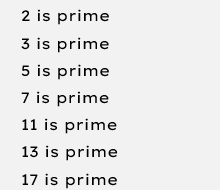The process continues until the prime number 97 is reached.

## Statements for loop control in Matlab

There is a control statement, which is a combination of conditions that decide whether the loop will be finished or not once the specified condition has become true. The control statement also determines how the loop will be structured. If you use a while loops in MATLAB, you define the comparative value(s) before the loop is initiated, whereas if you use a for loop, you define the value conditions when the loop starts.

The loop control statement enables you to control how the loop is executed and to deviate from the usual execution sequence. The loop control statements that are supported by MATLAB are the ‘break’ and ‘continue’ statements. Other languages as well support these commands.

### Break Statement

For or while loops are terminated by the break command. In the loop, all statements written after the break statement are skipped/ignored. When nested loops are encountered, break only exits the loop in which it is encountered.

### Statement of the case

Control is passed to the next loop iteration using the continue command. Similar to the break statement, the continue statement passes control to the next loop iteration. As opposed to terminating a loop, ‘continue’ allows the next iteration of the loop to start without any code in between.

## Final words

The purpose of this article is to introduce Loops in Matlab. MATLAB has a variety of loop types such as for loops, while loops, and nested loops that let the code handle looping requirements. In addition, these loops can be controlled by specific loop control statements. You can reduce the final code size by using loops for repetitive statements.